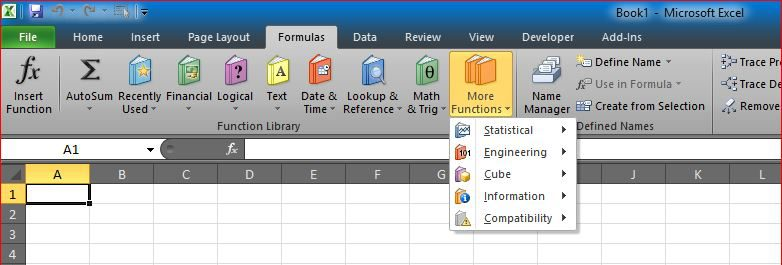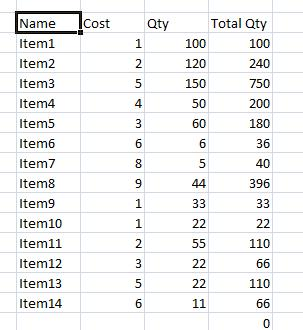# Rotate table using 1 formulaRotating table (Transpose) using functions so that the table is updated once the source table is updated.
Also can be used as automatically rotating tables with variable number of rows and columns

Sheet name can be used inside formula so that you can have multiple tables from different sheets to be rotate it into one sheet

The catch is that you need to know where is that table located and where you want to rotate it.

The best part is that it is a single formula to rotate the entire table including headers, here is the formula

`=INDIRECT(ADDRESS(COLUMN()+1,ROW()-6,,,"original table"))`